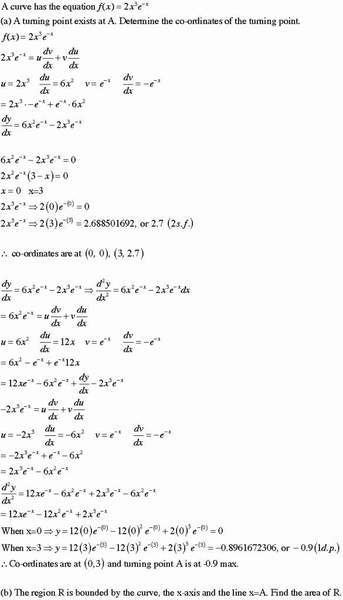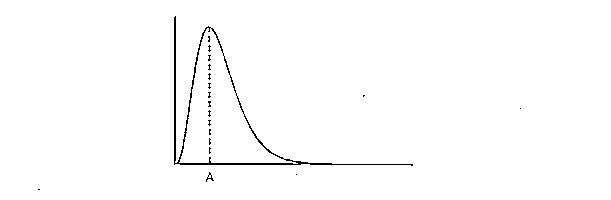# Q5A8-turning points and co-ordinates

• lemon

#### lemon

1. Hi. I have a part completed question. part a done. but not sure if correct. could someone check before i go onto part b please. thanks[/b

2. Homework Equations in image
3. The Attempt at a Solution in image#### Attachments

1. Hi. I have a part completed question. part a done. but not sure if correct. could someone check before i go onto part b please. thanks[/b

2. Homework Equations in image
3. The Attempt at a Solution in imageMuch of your work looks OK, but you have some stuff in there that shouldn't be there, and you arrived at an incorrect conclusion.

1. 2x3e-x = u dv/dx + v du/dx
Here you are confusing your original function with the formula for the product rule. In essence you are saying that your f(x) = the derivative of some product.

You should be saying something like d/dx(2x3e-x) = 6x2e-x - 2x3e-x

Here I used the product rule, but I don't show its formula. If you need to do that, put it off to the side.

2. When you evaluate your function at x = 0 and at x = 3, you have
2x3e-x ==> 2(0)e-0 = 0

and similar when you evaluate your function at x = 3.

The expression on the left is not a statement with a true or false value, so it can't imply anything.

Instead, you should write f(0) = 2(0)e0 = 0, and
f(3) = 2*33e-3 $\approx$ 2.6885

3. Following that you have
dy/dx = 6x2
e-x -2x3e-x ==> d2y/dx2 = 6x2
e-x -2x3e-xdx

First off, the second derivative is not equal to the first derivative. I know what you're trying to say, but how you're saying it is incorrect. Second, there should not be a dx at the end of that line.

You could say this instead: d2y/dx2 (or f''(x) = d/dx(6x2
e-x -2x3e-x), and then go ahead and calculate that derivative.

4. Following that you have more clutter similar to what I mentioned in #1, above. That shouldn't be there. You are also connecting a lot of expressions with = that aren't equal, and that's not good.

5. Finally, you conclude that "Co-ordinates are at (0, 3) and turning point A is at -0.9 max"

The point (0, 3) is not on the graph of your function, so you should not conclude that something important is going on there. The graph you show doesn't include anything for x < 0, which might be important.

Think back to what you did. At what x values was the first derivative 0? You're going to have turning points at those values, which you found to be (0, 0) and (3, ~2.7). Your second derivative is giving you information about the concavity of the graph of your function. You can use this information to specify where the turning point is.